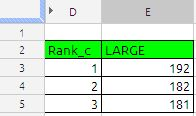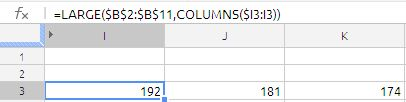## LARGE FUNCTION

The LARGE function returns the nth-largest value within a collection. The largest value is ranked number 1.

The Syntax of the function is

LARGE(data, rank_c)

data:  A collection of values. data may be a number,date,duration,set is a collection containing number, date, or duration values. All values must be of the same type.

rank_c:  A number representing the size ranking of the value you want to retrieve. ranking is a number value and must be in the range of 1 to the number of values in the collection.

Notes:

A ranking of 1 retrieves the largest number in the collection, 2 the second-largest, and so on. Values included in the array that are of the same size are ranked together, but impact the outcome.

We will try to understand the function with an example.

The Sample data set is as follows:if we want to identify the large three numbers of above data set  conventional way of writing the formula is as follows:The formula in cell E3 is

=LARGE(\$B\$2:\$B\$12,D3) and drag it down till E5 we will get the 1st largest 2nd largest and 3rd largest.

other way to extract largest 3 value with more robust formula is with use of ROWS function. the formula will be

=LARGE(\$B\$2:\$B\$11,ROWS(E\$3:E3)) and drag it down here we do not need a helped column D where argument rank_c.

Google Docs way of writing the formula is

=ArrayFormula(if(len(D3:D5);large(B2:B12,D3:D5);iferror(1/0)))

In the above formula you do not require to drag any thing the formula will automatically put 1st, 2nd and 3rd largest value in cell E3 to E5.

if we want to largest numbers across the columnsThe formula in cell I3 is

=LARGE(\$B\$2:\$B\$11,COLUMNS(\$I3:I3)) just drag it across.

if we want the sum of large three numbers in single cell,  formula will be as follows:

=sum(large(B2:B12;{1,2,3}))

which is nothing but sum of  192+182+181 = 555.

Advertisements